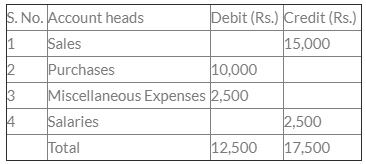# Test: Trial Balance

## 30 Questions MCQ Test Crash Course of Accountancy - Class 11 | Test: Trial Balance

Description
Attempt Test: Trial Balance | 30 questions in 30 minutes | Mock test for Commerce preparation | Free important questions MCQ to study Crash Course of Accountancy - Class 11 for Commerce Exam | Download free PDF with solutions
QUESTION: 1

Solution:
QUESTION: 2

Solution:

QUESTION: 3

### Trial Balance creates _______accuracy.

Solution:
QUESTION: 4

In trial balance, which accounts with normal balance are recorded at the credit side?

Solution:

Accrued expenses account with normal balance is shown at the credit side of a trial balance.

QUESTION: 5

Bhandari’s trial balance was showing difference of Rs. 5,000 (debit side exceeds). While checking of total sales register, he found that the total is overcast by Rs. 2,000. After correction in sales register what would be the difference in his trial balance.

Solution:
QUESTION: 6

Balances of the accounts are transferred to :

Solution:
QUESTION: 7

Difference of totals of both debit and credit side of trial balance is transferred to :

Solution:
QUESTION: 8

The preparation of trial balance is for :

Solution:
QUESTION: 9

After the preparation of ledgers, the next step is the preparation of

Solution:
QUESTION: 10

Methods of preparation of Trial Balance are :

Solution:
QUESTION: 11

Which of the following is not a process in the preparation of a Trial Balance ?

Solution:
QUESTION: 12

A Trial Balance contains the balances of :

Solution:
QUESTION: 13

The Trial Balance of M/S RAM & Co. shows closing Stock of Rs. 30,000. It will be recorded in :

Solution:
QUESTION: 14.

The difference in trial balance is due to:

Solution:
QUESTION: 15

A Trial balance will not balance if

Solution:
QUESTION: 16

Rs. 1500 received from sub-tenant for rent and entered correctly in the cash book is posted to the debit of the rent account. In the trial balance

Solution:

The correct option is A.

The correct journal entry should be

Cash Account         Dr.          1500

To Rent Account               1500

The entry in the cash book was correct, but the rent account was debited, which is incorrect because it should have been credited. Since the amount which has to be credited is not credited but instead is debited. So first the error is to be rectified, that is it will be credited to nullify the effect and then it will be credited again which should have been the correct entry. This means that the amount credited is doubled which will be 3000.

QUESTION: 17

Which of the following will not affect the agreement of Trial Balance ?

Solution:
QUESTION: 18

Methods of preparation of Trial Balance are :

Solution:
QUESTION: 19

Difference of totals of both debit and credit side of trial balance is transferred to :

Solution:
QUESTION: 20

__________ is prepare to ascertain the arithmetical accuracy of posting and balancing

Solution:
QUESTION: 21

A list which contains balances of accounts to know whether the debit and credit balances are matched.

Solution:
QUESTION: 22

Trial Balance under balance method is known as :

Solution:
QUESTION: 23

The preparation of trial balance is for :

Solution:
QUESTION: 24

After preparing the trial balance the accountant finds that the total of debit side is short by Rs. 1,500. This difference will be

Solution:
QUESTION: 25

Trial Balance is prepared on :

Solution:
QUESTION: 26

Agreement of Trial Balance is not a _______ proof of accuracy.

Solution:
QUESTION: 27

A Trial Balance contains the balances of :

Solution:
QUESTION: 28

Closing stock in the trial balance implies that.

Solution:
QUESTION: 29

Opening and Closing Balance of Debtors A/c were Rs. 30,000 and 40,000 respectively cash collected from the debtors during the year was Rs. 2,40,000. Discount allowed to debtors for timely payment amounted to Rs. 15,000 and bad debts written off were Rs. 10,00. Goods sold on credit were:

Solution:
QUESTION: 30

Which of the following in Trial Balance is contradictory to each other? __________.

Solution:

The correct option is D

A trial balance is the accounting equation of our business laid out in detail. It has our assets, expenses and drawings on the left (the debit side) and our liabilities, revenue and owner's equity on the right (the credit side).

Since inventory is an asset and drawings are expenses, both are debit items.

Sales are a form of income so go on the credit side of the trial balance.Purchases returns will reduce the expense so go on the credit side.

Carriage inwards in trial balance and Carriage outwards in trial balance are both treated as just another expense, so they are debit items

Trade receivables are revenues so are recorded on debit side and liability is recorded as a credit item.Use Code STAYHOME200 and get INR 200 additional OFF Use Coupon Code